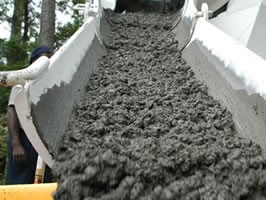# HOW TO FIND VOLUME OF CONCRETE PER BATCH?

## Aim

To determine volume of concrete per batch, where nominal maximum size of aggregate does not exceed 38 mm.

## Apparatus

Balance – Sensitive to 0.01 kg

Tamping bar – Length 38 cm, Weight 1.8 kg

Cylindrical mould – Dia = 250 mm, Height = 280 mm, Capacity = 0.01m3## Step by Step Procedure to Calculate Volume of Concrete per Batch

### Step-1 (Measuring Volume of Cylinder)

1. Fill the cylindrical mould with water at room temperature so that no meniscus is present above the rim.
2. Weigh the water required to fill the cylinder.
3. Divide the weight of water required to fill the mould by the unit weight of water, i.e. 1000 g/l. this will give the capacity (i.e. volume) of mould. Let it be “V1” in m3.

### Step-2 (Calculating Density of Compacted Concrete)

1. Fill fresh concrete into the mould in layers of about 5 cm deep and compact each layer by giving 60 strokes of tamping bar, distributed uniformly over cross-section of the mould.
2. Tap the exterior surface of the cylinder 10 to 15 times so that no air bubble appears on the surface of the compacted layer.
3. After compacting the concrete, use a flat cover plate to leave the mould just level fill.
4. Clean all the excess concrete from the exterior surface and weigh the mould with compacted concrete. Find out the weight of concrete by subtracting the weight of mould from the total weight. Let it be W1.
5. Calculate the weight per cubic meter of concrete (i.e. density of concrete) by dividing the weight of fully compacted concrete in the mould by the capacity of the measure (i.e. W1/V1).

Weight per cubic meter of concrete, W = W1/V1

Where,

W = Weight of concrete per cubic meter (i.e. Density of concrete), in kg/m3

W1 = Weight of the fully compacted concrete, in kg.

V1 = Volume of the cylindrical mould, in m3.

### Step-3 (Calculating Volume of Concrete per Batch)

Use the following formula to calculate volume of concrete per batch

V = [(N*50) + Wf + Wc + Ww] / W

Where,

V = Volume in m3 of concrete produced per batch

N = Number of 50 kg bags of cement per batch

Wf = Total weight in kg of fine aggregate per batch in condition used.

Wc = Total weight in kg of coarse aggregate per batch in condition used

Ww = Total weight in kg of mixing water added to batch

W = Density of concrete in kg/m3 (as calculated in step-2)